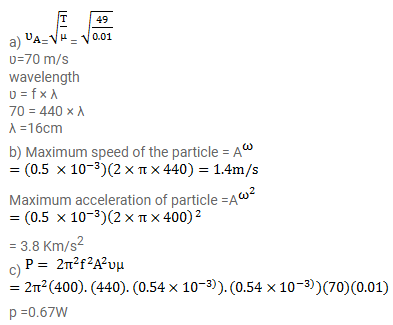# A tuning fork of frequency 400 Hz is attachedQuestion:

A tuning fork of frequency $400 \mathrm{~Hz}$ is attached to a long string of linear mass density $0.01 \mathrm{~kg} / \mathrm{m} \mathrm{kept}$ under a tension $49 \mathrm{~N}$. The fork produces transverse waves of amplitude 0-50 mm on the string.

(a) Find the wave speed and wavelength of the waves.

(b) Find the maximum speed and acceleration of a particle of the string.

(c) At what average rate is the tuning fork transmitting energy to the string?

Solution: